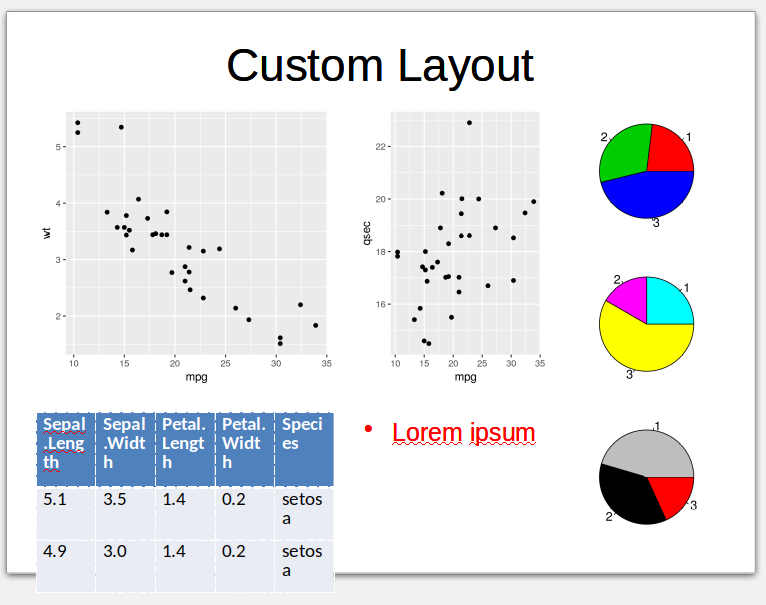customLayout is a simple extension of the basic layout function from R but it works not only with base and grid graphics systems, but also with PowerPoint slides using officer package.

## Instalation:

customLayout is available on CRAN:

install.packages("customLayout")

The development version can be installed from GitHub using devtools:

library(devtools)
install_github("zzawadz/customLayout")

## Basic functionality:

You can create layouts in the using the same syntax as in the base layout function:

library(customLayout)
lay <- lay_new(
matrix(1:4, nc = 2),
widths = c(3, 2),
heights = c(2, 1))
lay_show(lay)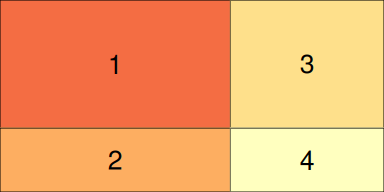lay2 <- lay_new(
matrix(1:4, nc = 2),
widths = c(3, 5),
heights = c(2, 4))
lay_show(lay2)But the main strength of this package is in combining created layouts with specific ratio:

# lay will be 3 times wider that lay2
# all ascects in lay and lay2 will be preserved
cl = lay_bind_col(lay, lay2, widths = c(3, 1))
lay_show(cl)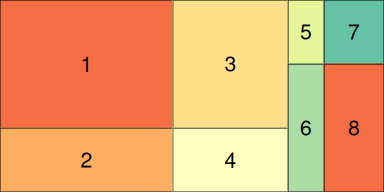You can create even more complicated layouts:

lay3 <- lay_new(matrix(1:2))
lay4 <- lay_bind_row(cl, lay3, heights = c(5, 2))
lay_show(lay4)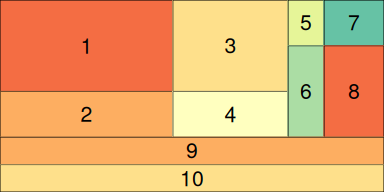lay5 <- lay_bind_col(lay4, lay3, widths = c(5, 2))
lay_show(lay5)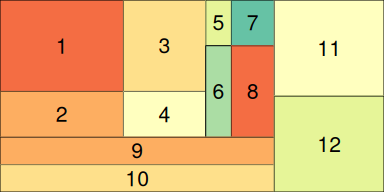## Split layout

You can create very complicated layouts by splitting one field:

library(customLayout)
lay <- lay_new(
matrix(1:4, nc = 2),
widths = c(3, 2),
heights = c(2, 1))
lay_show(lay)lay2 <- lay_new(
matrix(1:4, nc = 2),
widths = c(3, 5),
heights = c(2, 4))
lay_show(lay2)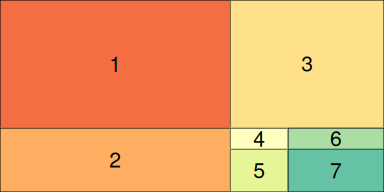# Split field 4 from lay into lay2:
slay <- lay_split_field(lay, lay2, field = 4)
lay_show(slay)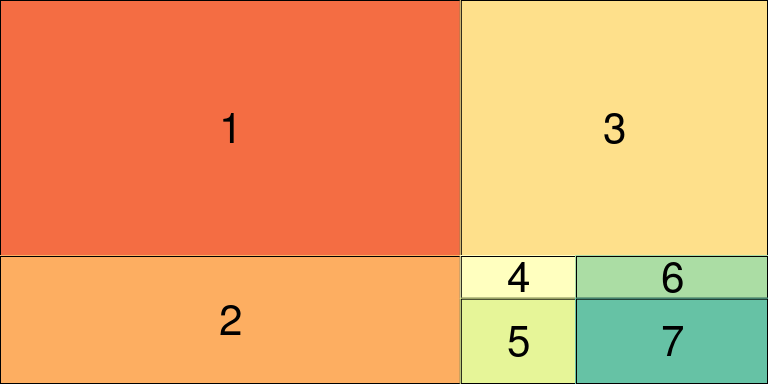## Example session:

### Base graphics

library(customLayout)
par(mar = c(3, 2, 2, 1))
lay  <- lay_new(
matrix(1:4, nc = 2),
widths = c(3, 2),
heights = c(2, 1))
lay2 <- lay_new(matrix(1:3))
cl   <- lay_bind_col(lay, lay2, widths = c(3, 1))

lay_set(cl) # initialize drawing area

set.seed(123)
plot(1:100 + rnorm(100))
plot(rnorm(100), type = "l")
hist(rnorm(500))
acf(rnorm(100))
pie(c(3, 4, 6), col = 2:4)
pie(c(3, 2, 7), col = 2:4 + 3)
pie(c(5, 4, 2), col = 2:4 + 6)## Grid graphics (ggplot2 and friends)

library(customLayout)
library(ggplot2)
library(gridExtra)

lay  <- lay_new(
matrix(1:2, ncol = 1))
lay2 <- lay_new(matrix(1:3))
cl   <- lay_bind_col(lay, lay2, widths = c(3, 1))

library(ggplot2)
cuts <- sort(unique(diamonds[["cut"]]),
decreasing = TRUE)

make_cut_plot <- function(cut) {
dd <- diamonds[diamonds[["cut"]] == cut, ]
pl <- ggplot(dd) +
geom_point(aes(carat, price)) +
facet_wrap("cut")
pl
}

plots <- lapply(cuts, make_cut_plot)

lay_grid(plots, cl)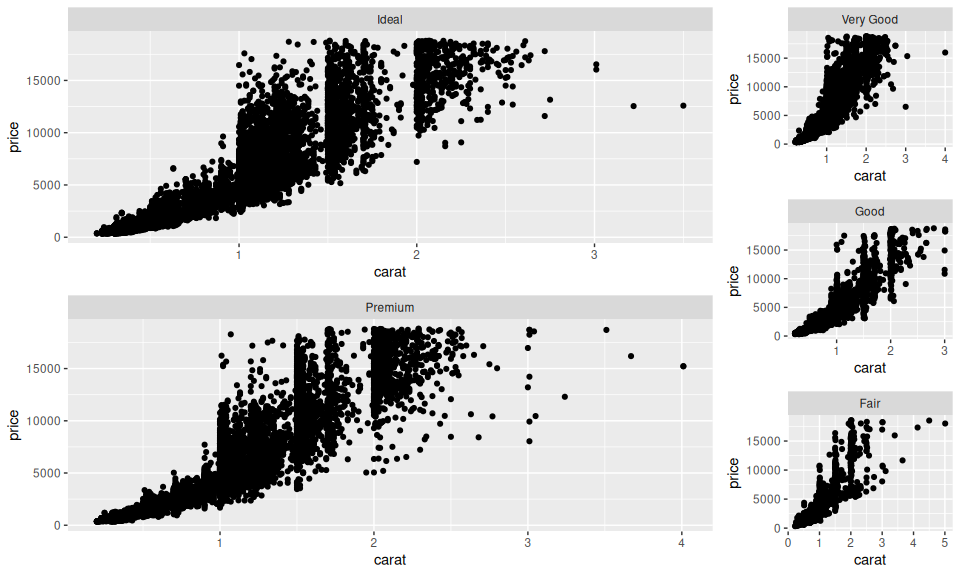## PowerPoint support

Layouts created using customLayout package can be used to arrange elements on the PowerPoint slide. For the detailed description please refer to the vignette:

vignette("layouts-for-officer-power-point-document", package = "customLayout")